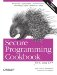# Comparing Floating-Point Numbers with Bounded Accuracy

Problem

You need to compare floating-point values, but you only want tests for equality, greater-than, or less-than to be concerned with a limited number of digits. For example, you want 3.33333 and 3.33333333 to show as being equal when comparing to a precision of .0001.

Solution

Write your own comparison functions that take a parameter that bounds the accuracy of the comparison. Example 3-6 shows the basic technique for such comparison functions.

Example 3-6. Comparing floating-point numbers

```#include
#include  // for fabs( )

using namespace std;

bool doubleEquals(double left, double right, double epsilon) {
return (fabs(left - right) < epsilon);
}

bool doubleLess(double left, double right, double epsilon,
bool orequal = false) {
if (fabs(left - right) < epsilon) {
// Within epsilon, so considered equal
return (orequal);
}
return (left < right);
}

bool doubleGreater(double left, double right, double epsilon,
bool orequal = false) {
if (fabs(left - right) < epsilon) {
// Within epsilon, so considered equal
return (orequal);
}
return (left > right);
}

int main( ) {

double first = 0.33333333;
double second = 1.0 / 3.0;
cout << first << endl;
cout << second << endl;

// Straight equalify test. Fails when you wouldn't want it to.
// (boolalpha prints booleans as "true" or "false")
cout << boolalpha << (first == second) << endl;
// New equality. Passes as scientific app probably wants.
cout << doubleEquals(first, second, .0001) << endl;
// New less-than
cout << doubleLess(first, second, .0001) << endl;
// New Greater-than
cout << doubleGreater(first, second, .0001) << endl;
// New less-than-or-equal-to
cout << doubleLess(first, second, .0001, true) << endl;
// New greater-than-or-equal-to
cout << doubleGreater(first, second, .0001, true) << endl;
}```

Following is the output from this example:

```0.333333
0.333333
false
true
false
false
true
true```

Discussion

The code in Example 3-6 starts with two values, 0.33333333 and whatever the computer figures 1.0 / 3.0 to be. It prints out the two values using the default formatting in cout; these two values appear to be the same at 0.333333. However, when you compare these two values, they are indeed different. The value of 1.0 / 3.0 has more significant digits than 0.33333333, and therefore, as far as your machine is concerned, the two numbers are not equal. In some applications, however, you may want these two numbers to show up as being the same.

The way to handle this is to write three of your own functions for comparing double values: doubleLess, doubleEquals, and doubleGreater, each of which takes two double values as parameters. Additionally, the doubleLess and doubleGreater take an additional parameter, which, when TRue, causes the functions to behave as less-than-or-equal or greater-than-or-equal, respectively.

To make these functions handle a precision, first consider the doubleEquals function. Instead of testing for equality, this function tests whether the difference between the two numbers is within a user-specified epsilon. (The example uses .0001 for the epsilon.) If so, then the function returns TRue, meaning the values are indeed the same. Thus, the values 0.3333, 0.33333, 0.333333, 0.33333333333, and 0.33333323438 would all show up as being equal.

To handle a less-than and greater-than operation, first test whether the numbers are equal within the range, as you did in the doubleEquals function. If so, then return true if you want to include equality in the test, and false if you don't. Otherwise, do a straight comparison.Secure Programming Cookbook for C and C++: Recipes for Cryptography, Authentication, Input Validation & More
ISBN: 0596003943
EAN: 2147483647
Year: 2006
Pages: 241